How Cheenta works to ensure student success?
Explore the Back-Story

# Number of Positive Divisors | Tomato objective 98This is an objective problem from TOMATO based on finding the Number of Positive Divisors.

Problem:

The number of positive integers which divide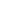is-
(A)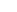; (B)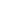; (C); (D);

Discussion:

We use the formula for computing number of divisors of a number:

Step 1: Prime factorise the given number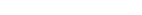Step 2: Use the formula for number of divisors: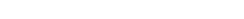Note:

Why this formula works? Basically, we are adding 1 to each exponent of each prime factor and then multiplying them. Refer to a discussion on Number Theoretic Functions (in any standard number theory book like David Burton's Elementary Number Theory).

This is an objective problem from TOMATO based on finding the Number of Positive Divisors.

Problem:

The number of positive integers which divideis-
(A); (B); (C); (D);

Discussion:

We use the formula for computing number of divisors of a number:

Step 1: Prime factorise the given numberStep 2: Use the formula for number of divisors:Note:

Why this formula works? Basically, we are adding 1 to each exponent of each prime factor and then multiplying them. Refer to a discussion on Number Theoretic Functions (in any standard number theory book like David Burton's Elementary Number Theory).

This site uses Akismet to reduce spam. Learn how your comment data is processed.

### 2 comments on “Number of Positive Divisors | Tomato objective 98”

1.Kaustav Roy says:

Sir, can you plzz suggest me how to do TOMATO OBJECTIVE NO. 99 .i.e. Sum of all positive divisors of 1800

1.Anish Ray says:

You can find the formula for the sum in any standard book namely An Excursion in Mathematics,Challenges and Thrills of Pre-College Mathematics.

Or you may surf up the google
Use the keyword-"Sum of divisors"

Hope this helps

### Knowledge Partner